# ME461: Quadcopter Control via Hand Tracking

OpenCV hand tracking to control the flight of a quadcopter via a TLV5610 DAC and MSP430 translation.

IntermediateShowcase (no instructions)20 hours257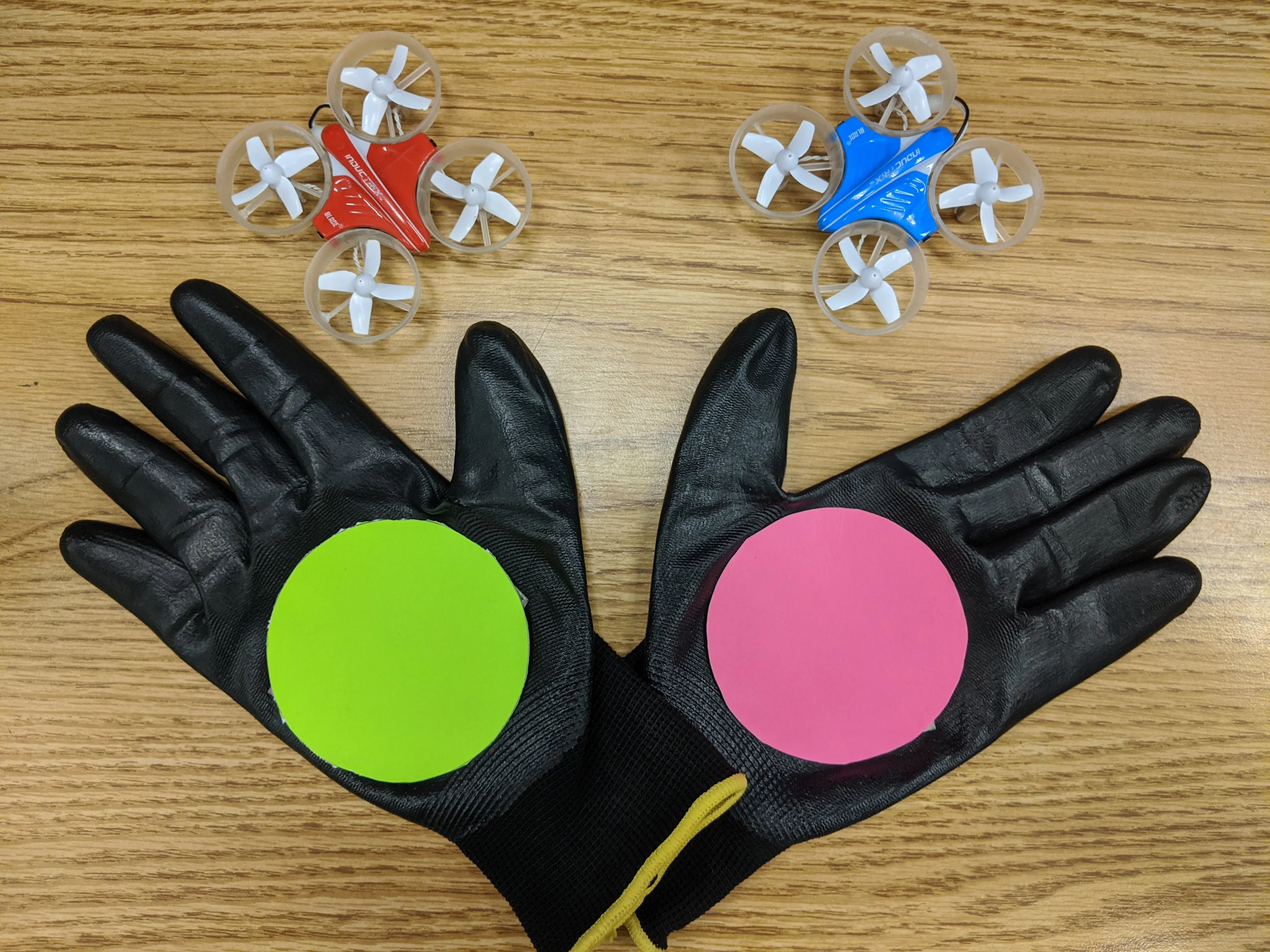## Things used in this project

### Hardware components

×1
 Texas Instruments MSP430 Greenboard
×1
 Texas Instruments TLV5610 DAC
×1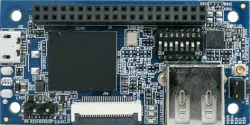96Boards Orange Pi i96
×1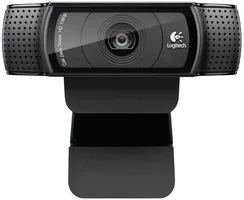Webcam, Logitech® HD Pro Any USB webcam will work.
×1

## Schematics

### Flight Test

Video taken during flight test, demonstrating control of the quad-copter by hand tracking.

### Circuit Images

Images of the circuitry used for copter control.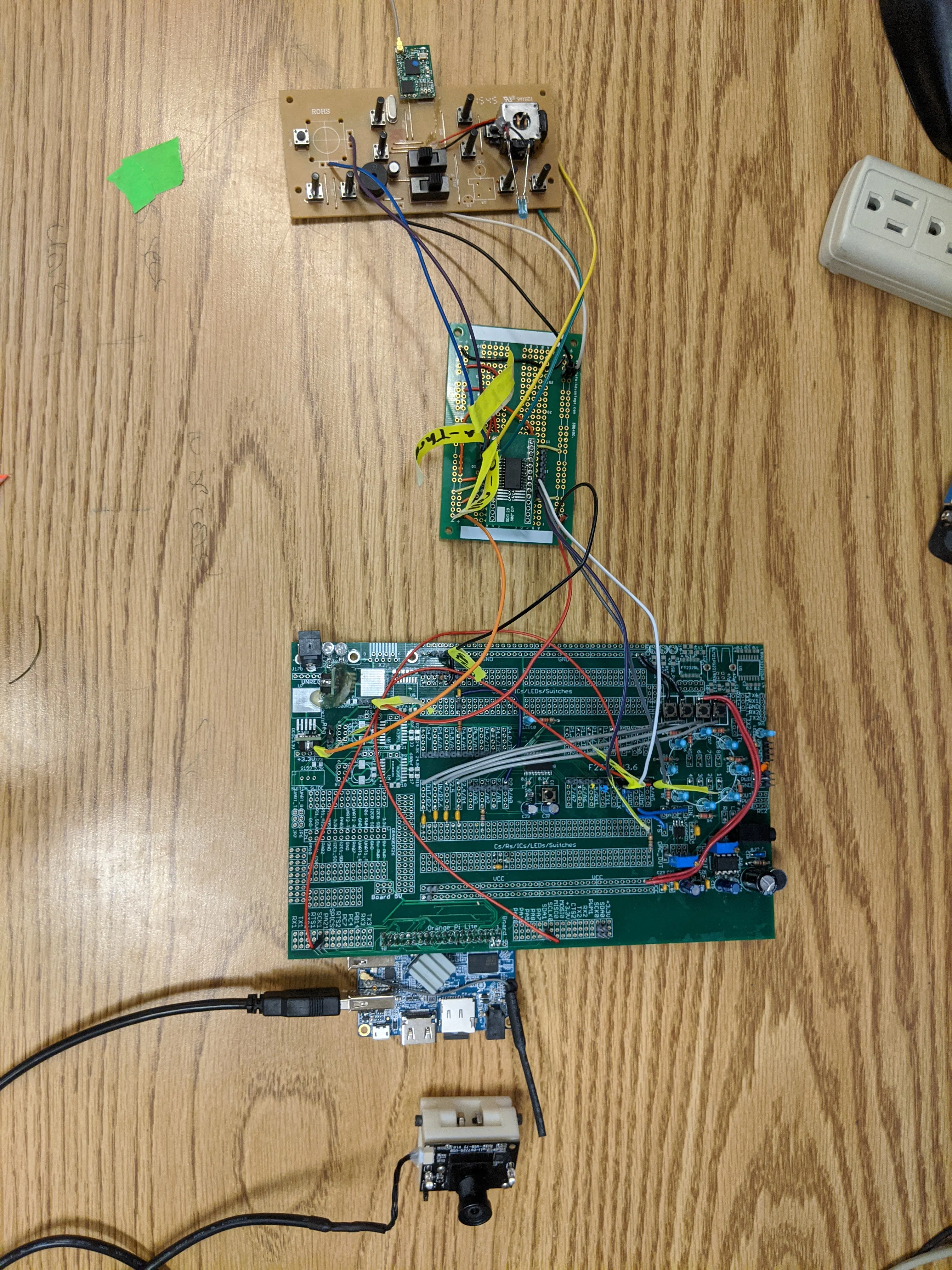Circuit developed to for the TLV5610ADC, a TI 2.7V to 5.5V 12-Bit 8-channel Serial Digital to Analog converter.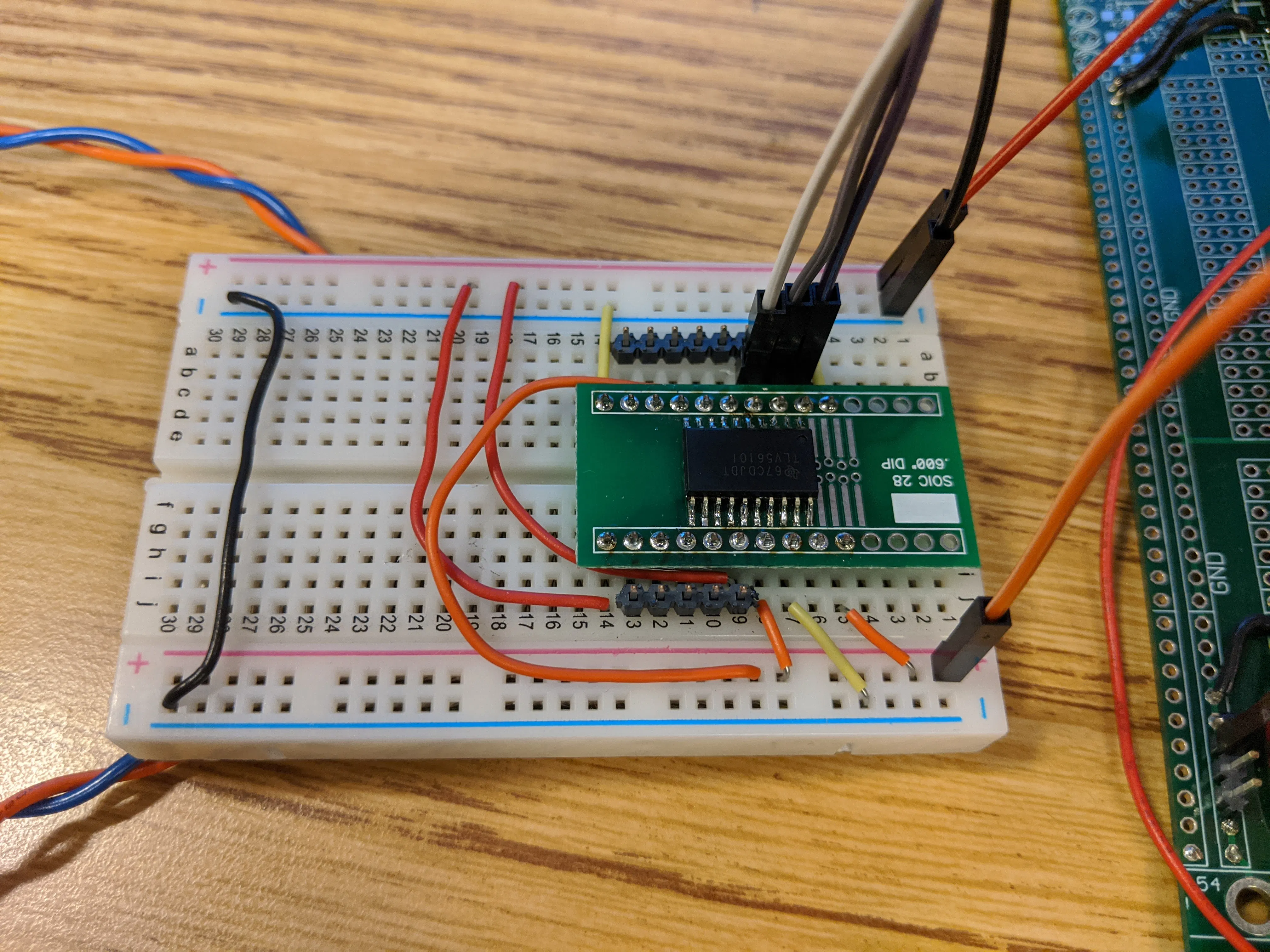## Code

### OrangePi Hand Tracking

```#include <stdio.h>
#include <opencv/cv.h>
#include <highgui.h>
#include <opencv2/videoio/videoio_c.h>
#include <math.h>
#include <sys/select.h>
#include <termios.h>
#include <stropts.h>
#include <unistd.h>
#include <fcntl.h>
#include <sys/ioctl.h>
#include <stdlib.h>
#include <stdint.h>
#include <sys/mman.h>
#include <linux/fb.h>
#include <time.h>

#define BILLION 1000000000L
#define I2C

#ifdef I2C
#include "i2c.h"

#endif

// Simple structure to hold details about the framebuffer device after it has
// been opened.
typedef struct {
// Pointer to the pixels. Try to not write off the end of it.
uint32_t * buffer;
// The file descriptor for the device.
int fd;
// Number of bytes in the buffer. To work out how many elements are in the buffer, divide this by 4 (i.e. sizeof(uint32_t))
size_t screen_byte_size;
// Structs providing further information about the buffer configuration. See https://www.kernel.org/doc/Documentation/fb/api.txt
struct fb_fix_screeninfo fix_info;
struct fb_var_screeninfo var_info;
} screen_t;

// Because you can't have too many variants of NULL
static const screen_t NULL_SCREEN = {0};
// Indicates if the passed screen_t struct is valid.
#define valid_screen(s) ((s).buffer != NULL)

#define NUMFRAME_ROWS 800
#define NUMFRAME_COLS 480
#define FRAMEOFFSET_ROWS 150
#define FRAMEOFFSET_COLS 30

#define NUM_ROWS 120
#define NUM_COLS 160

screen_t open_fb(void);
void close_fb(screen_t *screen);
int my_min(int a, int b, int c);
int my_max(int a, int b, int c);
void rgb2hsv(int red,int green,int blue,int *hue,int *sat,int *value);
int _kbhit(void);

unsigned int i = 0;
//Our variables
unsigned int numpixels = 0;
unsigned int rowsum = 0;
unsigned int colsum = 0;

unsigned int numpixels2 = 0;
unsigned int rowsum2 = 0;
unsigned int colsum2 = 0;

unsigned int center_r = 0;
unsigned int center_c = 0;
unsigned int ave_center_r = 0;
unsigned int ave_center_c = 0;
unsigned long ave_center_c_sum = 0;
unsigned long ave_center_r_sum = 0;

unsigned int length_ave = 50;

unsigned int center_r2 = 0;
unsigned int center_c2 = 0;
unsigned int ave_center_r2 = 0;
unsigned int ave_center_c2 = 0;
unsigned long ave_center_c2_sum = 0;
unsigned long ave_center_r2_sum = 0;

int throt_dist = 0; //Throttle Distance
int yaw_dist = 0; //Yaw Distance
int pitch_dist = 0; //Pitch Dist
int roll_dist = 0; //Roll Dist

//Control Output Vars
int throt_val = 0;
int yaw_val = 0;
int pitch_val = 0;
int roll_val = 0;

signed int throt_comp = 0;
signed int yaw_comp = 0;
signed int pitch_comp = 0;
signed int roll_comp = 0;

unsigned int pixel_duty = 3; //Number of duty per pixel

int main(void) {
#ifdef I2C
int bus;
// add any other I2C variables here
unsigned char tx;
unsigned char rx;
unsigned int tx_1 = 0, tx_2 = 0, tx_3 = 0, tx_4 = 0;
unsigned int rx_1 = 0, rx_2 = 0, rx_3 = 0, rx_4 = 0;
#endif

uint64_t diff;
struct timespec current_time,previous_time;

int key=0;
CvCapture* capture;

IplImage *frame, *frame_hsv;

capture= cvCaptureFromCAM(-1);

cvSetCaptureProperty(capture,CV_CAP_PROP_FRAME_WIDTH, NUM_COLS);  // Number of columns
cvSetCaptureProperty(capture,CV_CAP_PROP_FRAME_HEIGHT, NUM_ROWS); // Number of rows

if(!capture) printf("No camera detected \n");

frame=cvQueryFrame(capture);
frame_hsv=cvCreateImage(cvGetSize(frame),8,3);

#ifdef I2C
bus = i2c_start_bus(1);
#endif

screen_t screen = open_fb();
if (!valid_screen(screen)) {
printf("ERROR: Failed to open screen\n");
return 1;
}

unsigned int arr_center_c[length_ave];
unsigned int arr_center_r[length_ave];
unsigned int arr_center_c2[length_ave];
unsigned int arr_center_r2[length_ave];

float period=0,Hz=0;
unsigned char *data_hsv, *data_bgr, *current_pixel;
int r=0;
int c=0;
int nr;
int nc;
int celements;
nr=frame_hsv->height; //number of rows in image should be 120
nc=frame_hsv->width; //number of collumns
celements = nc*3;  // b,g,r elements in each column

int vh=255, vl=166, sh=255, sl=118, hh=230, hl=20;
int h=0, s=0, v=0;
int blue=0, green=0, red=0;

int vh2=255, vl2=90, sh2=255, sl2=120, hh2=138, hl2=104;

clock_gettime(CLOCK_MONOTONIC, &previous_time);

while(! _kbhit()) {  //27 is the code corresponding to escape key

frame=cvQueryFrame(capture);

if(!frame) break;

// -----loop through image pixels-----
data_bgr=(unsigned char *)(frame->imageData);
for(r=0; r<nr; r++) {
for(c=0; c<nc;c++) {
blue = (int)data_bgr[r*celements+c*3];
green = (int)data_bgr[r*celements+c*3+1];
red = (int)data_bgr[r*celements+c*3+2];
rgb2hsv(red,green,blue,&h,&s,&v);

//--------------process each thresholded pixel here------------------
if( (h <=hl || h >= hh) && (s>=sl && s<=sh) && (v>=vl && v<=vh) ) {
green = 0;
blue = 255;
red = 0;

numpixels++;
rowsum = rowsum + r;
colsum = colsum + c;

}

else if( (h >=hl2 && h <= hh2) && (s>=sl2 && s<=sh2) && (v>=vl2 && v<=vh2) ) {
green = 0;
blue = 255;
red = 255;

numpixels2++;
rowsum2 = rowsum2 + r;
colsum2 = colsum2 + c;

}

screen.buffer[(r+FRAMEOFFSET_ROWS)*NUMFRAME_COLS + (FRAMEOFFSET_COLS + c)] = (int)((red<<16) | (green<<8) | blue);

}
} //--------------end pixel processing---------------------
if (numpixels > 20){
center_c = colsum  / numpixels;
center_r = rowsum  / numpixels;

for (i = 1; i<length_ave; i++ ){
arr_center_c[i-1] = arr_center_c[i];
arr_center_r[i-1] = arr_center_r[i];
arr_center_c = center_c;
arr_center_r = center_r;
}

//data_bgr(centerx * celement + centery * 3) = [255, 255, 255];
}

if (numpixels2 > 20){
center_c2 = colsum2 / numpixels2;
center_r2 = rowsum2 / numpixels2;

for (i = 1; i<length_ave; i++ ){
arr_center_c2[i-1] = arr_center_c2[i];
arr_center_r2[i-1] = arr_center_r2[i];
arr_center_c2 = center_c2;
arr_center_r2 = center_r2;
}

}

ave_center_c_sum = 0;
ave_center_r_sum = 0;
ave_center_c2_sum = 0;
ave_center_r2_sum = 0;
for (i = 0; i < length_ave; i++){
ave_center_c_sum += arr_center_c[i];
ave_center_r_sum += arr_center_r[i];
ave_center_c2_sum += arr_center_c2[i];
ave_center_r2_sum += arr_center_r2[i];
}
ave_center_c = ave_center_c_sum / length_ave;
ave_center_r = ave_center_r_sum / length_ave;
ave_center_c2 = ave_center_c2_sum / length_ave;
ave_center_r2 = ave_center_r2_sum / length_ave;

throt_dist = 120 - ave_center_r;

yaw_dist = ave_center_c - center_c;
pitch_dist = ave_center_r2- center_r2 ;
roll_dist = ave_center_c2 - center_c2;

throt_dist += 50;
yaw_dist += 50;
pitch_dist += 50;
roll_dist += 50;

throt_comp = throt_dist * 512  * 2 / 100;
yaw_comp = yaw_dist * 512 * pixel_duty / 100;
pitch_comp = pitch_dist * 512 * pixel_duty / 100;
roll_comp = roll_dist * 512 * pixel_duty / 100;

throt_val = throt_comp - (50 * 512 * 2)/100;
yaw_val = 512 + yaw_comp - (50 * 512 * pixel_duty)/100;
pitch_val = 512 + pitch_comp - (50 * 512 * 2)/100;
roll_val = 512 + roll_comp - (50 * 512 * 2)/100;

if (throt_val < 0){ throt_val = 0;} else if (throt_val > 1023){ throt_val = 1023;}
if (yaw_val < 0){ yaw_val = 0;} else if (yaw_val > 1023){ yaw_val = 1023;}
if (pitch_val < 0){ pitch_val = 0;} else if (pitch_val > 1023){ pitch_val = 1023;}
if (roll_val < 0){ roll_val = 0;} else if (roll_val > 1023){ roll_val = 1023;}

if (numpixels < 150){
throt_val = 0;
yaw_val = 512;
}

if (numpixels2 < 100){
pitch_val = 512;
roll_val = 512;
}

//system("clear");
printf("Thrt Yaw  Ptch Roll Pix1 Pix2\n\r");

//printf("%4d %4d %4d %4d\n\r",ave_center_r, ave_center_c, ave_center_r2, ave_center_c2);
//printf("%3d %3d %3d %3d \n\r", throt_dist, yaw_dist, pitch_dist, roll_dist);
printf("%4d %4d %4d %4d %4d %4d \n\r", throt_val, yaw_val, pitch_val, roll_val, numpixels, numpixels2);
//printf("%4d %4d %4d\r\n", throt_dist, throt_val, throt_comp);

tx_1 = throt_val;
tx_2 = yaw_val;
tx_3 = pitch_val;
tx_4 = roll_val;

/*
tx_1 = 1;
tx_2 = 2;
tx_3 = 3;
tx_4 = 4;
*/
tx = tx_1 >> 8;
tx = tx_1;
tx = tx_2 >> 8;
tx = tx_2;
tx = tx_3 >> 8;
tx = tx_3;
tx = tx_4 >> 8;
tx = tx_4;

rx_1 = (rx << 8 )+ rx;
rx_2 = (rx << 8 )+ rx;
rx_3 = (rx << 8 )+ rx;
rx_4 = (rx << 8 )+ rx;

printf("%4d %4d %4d %4d \n\r", rx_1, rx_2, rx_3, rx_4);
//rx_long1 = (((long)rx) << 24) + (((long)rx) << 16) + (((long)rx) << 8) + ((long)rx);
//rx_long2 = (((long)rx) << 24) + (((long)rx) << 16) + (((long)rx) << 8) + ((long)rx);

//usleep(200*1000);

/*
screen.buffer[(center_r+FRAMEOFFSET_ROWS)*NUMFRAME_COLS + (FRAMEOFFSET_COLS + center_c)] = (int)((red<<16) | (green<<8) | blue);
screen.buffer[(1 + center_r+FRAMEOFFSET_ROWS)*NUMFRAME_COLS + (FRAMEOFFSET_COLS + center_c)] = (int)((red<<16) | (green<<8) | blue);
screen.buffer[(-1+ center_r+FRAMEOFFSET_ROWS)*NUMFRAME_COLS + (FRAMEOFFSET_COLS + center_c)] = (int)((red<<16) | (green<<8) | blue);
screen.buffer[(center_r+FRAMEOFFSET_ROWS)*NUMFRAME_COLS + (FRAMEOFFSET_COLS + center_c + 1 )] = (int)((red<<16) | (green<<8) | blue);
screen.buffer[(center_r+FRAMEOFFSET_ROWS)*NUMFRAME_COLS + (FRAMEOFFSET_COLS + center_c - 1)] = (int)((red<<16) | (green<<8) | blue);
*/

numpixels = 0;
colsum = 0;
rowsum = 0;

numpixels2 = 0;
colsum2 = 0;
rowsum2 = 0;

clock_gettime(CLOCK_MONOTONIC, &current_time);
diff = BILLION * (current_time.tv_sec - previous_time.tv_sec) + current_time.tv_nsec - previous_time.tv_nsec;
period = diff * 1e-9;
if (period > 0.0) {
Hz = 1.0/period;
} else {
Hz = 0.0;
}
//printf("Hz = %.4f\n",Hz);
previous_time = current_time;
}  // end while

cvReleaseCapture(&capture);

#ifdef I2C
i2c_close_bus(bus);
#endif

printf("All done, bye bye.\n");
close_fb(&screen);

return 0;
}

int my_min(int a, int b, int c) {

int min;
min = a;

if (b < min) {
min = b;
}
if (c < min) {
min = c;
}
return (min);
}

int my_max(int a, int b, int c) {
int max;
max = a;
if (b > max) {
max = b;
}
if (c > max) {
max = c;
}
return (max);
}

void rgb2hsv(int red,int green,int blue,int *hue,int *sat,int *value) {
int min, delta;
min = my_min( red, green, blue );

*value = my_max( red, green, blue );

delta = *value - min;

if( *value != 0 ) {

*sat = (delta*255) / *value; // s

if (delta != 0) {

if( red == *value )
*hue = 60*( green - blue ) / delta; // between yellow & magenta

else if( green == *value )
*hue = 120 + 60*( blue - red ) / delta;  // between cyan & yellow
else
*hue = 240 + 60*( red - green ) / delta; // between magenta & cyan

if( *hue < 0 )
*hue += 360;

} else {
*hue = 0;
*sat = 0;
}

} else {
// r = g = b = 0
// s = 0, v is undefined
*sat = 0;
*hue = 0;
}

*hue = (*hue*255)/360;

}

/**
* Opens the first frame buffer device and returns a screen_t struct
* for accessing it. If the framebuffer isn't in the expected format
* (32 bits per pixel), NULL_SCREEN will be returned.
*/
screen_t open_fb(void) {
const char * const SCREEN_DEVICE = "/dev/fb0";
int screen_fd = open(SCREEN_DEVICE, O_RDWR);
if (screen_fd == -1) {
printf("ERROR: Failed to open %s\n", SCREEN_DEVICE);
return NULL_SCREEN;
}

struct fb_var_screeninfo var_info = {0};
if (ioctl(screen_fd, FBIOGET_VSCREENINFO, &var_info) == -1) {
printf("ERROR: Failed to get variable screen info\n");
return NULL_SCREEN;
}

struct fb_fix_screeninfo fix_info = {0};
if (ioctl(screen_fd, FBIOGET_FSCREENINFO, &fix_info) == -1) {
printf("ERROR: Failed to get fixed screen info\n");
return NULL_SCREEN;
}

if (var_info.bits_per_pixel != 32) {
printf("ERROR: Only support 32 bits per pixel. Detected bits per pixel: %d\n", var_info.bits_per_pixel);
return NULL_SCREEN;
}

const size_t screen_byte_size = var_info.xres * var_info.yres * var_info.bits_per_pixel / 8;
uint32_t * const buffer = (uint32_t *)mmap(NULL, screen_byte_size, PROT_READ | PROT_WRITE, MAP_SHARED, screen_fd, 0 /* offset */);

screen_t screen = {
.buffer = buffer,
.fd = screen_fd,
.screen_byte_size = screen_byte_size,
.fix_info = fix_info,
.var_info = var_info
};
return screen;
}

/**
* Closes the framebuffer when you are finished with it. Don't try
* to access things in the struct after calling this or else a
* kitten will die.
*/
void close_fb(screen_t *screen) {
munmap(screen->buffer, screen->screen_byte_size);
close(screen->fd);
*screen = NULL_SCREEN;
}

int _kbhit() {
static const int STDIN = 0;
static int initialized = 0;

if (! initialized) {
// Use termios to turn off line buffering
struct termios term;
tcgetattr(STDIN, &term);
term.c_lflag &= ~ICANON;
tcsetattr(STDIN, TCSANOW, &term);
setbuf(stdin, NULL);
initialized = 1;
}

int bytesWaiting;
return bytesWaiting;
}
```

### MSP430 I2C to DAC Conversion

```/******************************************************************************
MSP430F2272 Project Creator 4.0

ME 461 - S. R. Platt
Fall 2010

Updated for CCSv4.2 Rick Rekoske 8/10/2011

Written by: Steve Keres
College of Engineering Control Systems Lab
University of Illinois at Urbana-Champaign
*******************************************************************************/

#include "msp430x22x2.h"
#include "UART.h"

char newprint = 0;
unsigned long timecnt = 0;
unsigned long timecnt2 = 0;
unsigned char RXData = {0,0,0,0,0,0,0,0};
unsigned char TXData = {0,0,0,0,0,0,0,0};
int I2Cindex = 0;
int rx1=0,rx2=0,rx3=0,rx4=0;
int tx1 = 0, tx2 = 0, tx3 = 0, tx4 = 0;
//long I2C_rx1=0, I2C_rx2=0, I2C_rx3=0, I2C_rx4=0;
//const long I2C_tx1=0, I2C_tx2=0, I2C_tx3=0, I2C_tx4=0;
int switch_state = 0;
unsigned int kill = 0;

signed int throt_val = 0;
signed int throt_trim = 0;
unsigned int trim = 0;

unsigned int yaw_val = 2048;
unsigned int pitch_val = 2048;
unsigned int roll_val = 2048;

unsigned int CTRL0 = 0;
unsigned int CTRL1 = 0;

unsigned int DAC_data = 0;
unsigned int msb_flag = 0;
char LSB_data = 0;
char MSB_data = 0;
unsigned int byte_count = 0;

unsigned int button_count_1 = 0;
unsigned int button_count_2 = 0;
unsigned int button_count_3 = 0;
unsigned int button_count_4 = 0;

char get_switch_state(void){
//Strictly looking at state of p2in, for ports 4-7
//Make sure to note high vs low = on vs off for individual ports
switch_state = 0;
//Port 7
if ((P2IN & 0x80) == 0){
//If true, switch 4 pressed
switch_state |= 0x8;
}
if ((P2IN & 0x40) == 0){
//If True, switch 3 pressed
switch_state |= 0x4;
}
if ((P2IN & 0x20) == 0x20){
//If True, switch 2 pressed
switch_state |= 0x2;
}
if ((P2IN & 0x10) == 0x10){
//If True, switch 1 pressed
switch_state |= 0x1;
}

return switch_state;
}

void DAC_write(int DAC_data){
//DAC_data = DAC_data_uform;
//DAC_data = DAC_data << 2;
//DAC_data |= 0x4000;

LSB_data = DAC_data;
MSB_data = DAC_data>>8;

//Trigger FS pin high to low
P3OUT |= 0x08;
P3OUT &= ~0x08;

//Fill most significant
msb_flag = 1; //Data just filled buffer
UCA0TXBUF = MSB_data;
}

void main(void) {

WDTCTL = WDTPW + WDTHOLD;                 // Stop WDT

if (CALBC1_16MHZ ==0xFF || CALDCO_16MHZ == 0xFF) while(1);

DCOCTL = CALDCO_16MHZ;                      // Set uC to run at approximately 16 Mhz
BCSCTL1 = CALBC1_16MHZ;

//P1IN              Port 1 register used to read Port 1 pins setup as Inputs
P1SEL &= ~0xFF; // Set all Port 1 pins to Port I/O function
P1REN &= ~0xFF; // Disable internal resistor for all Port 1 pins
P1DIR |= 0x3;  // Set Port 1 Pin 0 and Pin 1 (P1.0,P1.1) as an output.  Leaves Port1 pin 2 through 7 unchanged
P1OUT &= ~0xFF;  // Initially set all Port 1 pins set as Outputs to zero
P1IFG &= ~0xFF;  // Clear Port 1 interrupt flags
P1IES &= ~0xFF; // If port interrupts are enabled a High to Low transition on a Port pin will cause an interrupt
P1IE &= ~0xFF; // Disable all port interrupts

//Output for LED

//    P3SEL &= ~BIT0;
//    P3REN &= ~BIT0;
//    P3DIR |= BIT0;
//    P3OUT &= ~BIT0;

P3SEL |= BIT1 + BIT2;          // Assign I2C pins to USCI_B0

UCB0CTL1 = UCSSEL_2 + UCSWRST;           // source SMCLK, hold USCIB0 in reset

UCB0CTL0 = UCMODE_3 + UCSYNC; // I2C Slave, synchronous mode
UCB0I2COA = 0x25;             // Own Address is 0x25
UCB0CTL1 &= ~UCSWRST;         // Clear SW reset, resume operation
IE2 |= UCB0RXIE;              // Enable RX interrupt only.  TX will be enabled after reception of an I2C RX

/*

*/
//Port 2 Configuration Section
//P2IN          Port 2 register used to read Port 2 pins setup as Inputs
P2SEL = 0x00; // Set all Port 2 pins to Port I/O function
P2REN = 0xF0; // 1111 0000 Enable internal resistor for all Port 2.4-2.7
P2DIR = 0x00; // Set Port 2 Pins to input only
P2OUT = 0xC0; //1100 0000 Set P2.6-P2.7 to pull up

P2IFG = 0x00; //Clears all initial interrupts
P2IES = 0xC0; //Set P2.6-P2.7 high to low, P2.4-P2.5 low to high
P2IE  = 0xF0; //Enable all interrupts

// Timer A Config
TACCTL0 = CCIE;                           // Enable Timer A interrupt
TACCR0 = 16000;                           // period = 1ms
TACTL = TASSEL_2 + MC_1;                  // source SMCLK, up mode

//DAC Config

CTRL0 |= 0x8008;
CTRL1 |= 0x9000;

UCA0CTL0 = UCCKPH + UCCKPL + UCMSB + UCMST + UCMODE_0 + UCSYNC;
UCA0CTL1 = UCSSEL_2 + UCSWRST;
UCA0BR0 = 0x10;
UCA0BR1 = 0x00;
UCA0CTL1 &= ~UCSWRST;
IE2 |= UCA0RXIE;
IFG2 &= ~UCA0RXIFG;
//P3SEL &= ~0X18;
//P3SEL |= 0X01;
//P3DIR |= 0X18;

P3SEL &= ~0x08;
P3DIR |= 0x08;

P3SEL |= 0x01;

P3SEL |= 0x10;

//Init_UART(115200,1);    // Initialize UART for 115200 baud serial communication

_BIS_SR(GIE);   // Enable global interrupt

DAC_write(CTRL0);
DAC_write(CTRL1);
while(1) {

if(newmsg) {
//my_scanf(rxbuff,&var1,&var2,&var3,&var4);
newmsg = 0;
}

if (newprint)  {

//UART_printf("R:yourRXdata\n\r");
// UART_send(1,(float)timecnt/500);
newprint = 0;
}

}
}

// Timer A0 interrupt service routine
#pragma vector=TIMERA0_VECTOR
__interrupt void Timer_A (void)
{
timecnt++; // Keep track of time for main while loop.

if (timecnt >= 10){
DAC_write(throt_val);
timecnt = 0;
}

if (timecnt2 >= 500){
newprint = 1;  // flag main while loop that .5 seconds have gone by.
}

if (timecnt2 >= 500){
button_count_1 = 0;
button_count_2 = 0;
button_count_3 = 0;
button_count_4 = 0;
}

}

/*
// ADC 10 ISR - Called when a sequence of conversions (A7-A0) have completed

tx1 = 1;
tx2 = 2;
tx3 = 3;
tx4 = 4;

TXData = tx1>>8;
TXData = tx1;
TXData = tx2>>8;
TXData = tx2;
TXData = tx3>>8;
TXData = tx3;
TXData = tx4>>8;
TXData = tx4;

P3OUT ^= BIT0;
newprint = 1;
IE2 &= ~UCB0RXIE;
IE2 |= UCB0TXIE;

}
*/

// USCI Transmit ISR - Called when TXBUF is empty (ready to accept another character)
#pragma vector=USCIAB0TX_VECTOR
__interrupt void USCI0TX_ISR(void) {

if((IFG2&UCA0TXIFG) && (IE2&UCA0TXIE)) {        // USCI_A0 requested TX interrupt
if(printf_flag) {
if (currentindex == txcount) {
senddone = 1;
printf_flag = 0;
IFG2 &= ~UCA0TXIFG;
} else {
UCA0TXBUF = printbuff[currentindex];
currentindex++;
}
} else if(UART_flag) {
if(!donesending) {
UCA0TXBUF = txbuff[txindex];
if(txbuff[txindex] == 255) {
donesending = 1;
txindex = 0;
} else {
txindex++;
}
}
}

IFG2 &= ~UCA0TXIFG;
}

if((IFG2&UCB0RXIFG) && (IE2&UCB0RXIE)) {    // USCI_B0 RX interrupt occurs here for I2C
RXData[I2Cindex] = UCB0RXBUF;           // Read RX buffer

I2Cindex++;
if (I2Cindex == 8) {
rx1 = (((int)RXData)<<8) + ((int)RXData);
rx2 = (((int)RXData)<<8) + ((int)RXData);
rx3 = (((int)RXData)<<8) + ((int)RXData);
rx4 = (((int)RXData)<<8) + ((int)RXData);

I2Cindex = 0;
IE2 &= ~UCB0RXIE;

TXData = rx1>>8;
TXData = rx1;
TXData = rx2>>8;
TXData = rx2;
TXData = rx3>>8;
TXData = rx3;
TXData = rx4>>8;
TXData = rx4;

IE2 &= ~UCB0RXIE;
IE2 |= UCB0TXIE;

throt_val = rx1 * 4;
//throt_val = 4095;
yaw_val = rx2 * 4;
pitch_val = rx3 * 4;
roll_val = rx4 * 4;

throt_val += throt_trim;

if (throt_val > 4095){
throt_val = 4095;
}
if (throt_val < 5){
throt_val = 2;
}
/*
while (kill == 1){
throt_val = 0;
yaw_val = 2048;
pitch_val = 2048;
roll_val = 2048;

}*/

throt_val |= 0x0000;
//throt_val |= 0x0FFF; //full throttle for testing
yaw_val |= 0x1000;
pitch_val |= 0x2000;
roll_val |= 0x3000;

}

} else if ((IFG2&UCB0TXIFG) && (IE2&UCB0TXIE)) { // USCI_B0 TX interrupt
UCB0TXBUF = TXData[I2Cindex];
I2Cindex++;
if (I2Cindex == 8) {
I2Cindex=0;
IE2 &= ~UCB0TXIE;
IE2 |= UCB0RXIE;
}
}
}

#pragma vector=PORT2_VECTOR
__interrupt void Port_2(void)
{

if ((P2IFG & 0x10) == 0x10){
if (button_count_1 >= 1){
}
else{

button_count_1 += 1;

if (kill == 0){
kill = 1;
}
else{
kill = 0;
}
}

}

if ((P2IFG & 0x20) == 0x20){
if (button_count_2 >= 1){
}
else{
button_count_2 +=1;

throt_trim += trim;
}
}

if ((P2IFG & 0x40) == 0x40){
if (button_count_3 >= 1){
}
else{
button_count_3 += 1;
throt_trim -= trim;
}
}

}

// USCI Receive ISR - Called when shift register has been transferred to RXBUF
// Indicates completion of TX/RX operation
#pragma vector=USCIAB0RX_VECTOR
__interrupt void USCI0RX_ISR(void) {

if((IFG2&UCA0RXIFG) && (IE2&UCA0RXIE)) {  // USCI_A0 requested RX interrupt (UCA0RXBUF is full)
if (msb_flag == 1){
UCA0TXBUF = LSB_data; //Transmit LSB data
msb_flag = 0; //Set flag to 0 to prevent loop of empty data
byte_count++;
}
else{
if (byte_count == 1){
DAC_write(yaw_val);
}
else if (byte_count == 2){
DAC_write(pitch_val);
}
else if (byte_count == 3){
DAC_write(roll_val);
}
else if (byte_count == 4){
byte_count = 0;
}
}
IFG2 &= ~UCA0RXIFG;
}

if((UCB0I2CIE&UCNACKIE) && (UCB0STAT&UCNACKIFG)) { // I2C NACK interrupt

UCB0STAT &= ~UCNACKIFG;
}
if((UCB0I2CIE&UCSTPIE) && (UCB0STAT&UCSTPIFG)) { // I2C Stop interrupt

UCB0STAT &= ~UCSTPIFG;
}
if((UCB0I2CIE&UCSTTIE) && (UCB0STAT&UCSTTIFG)) { //  I2C Start interrupt

UCB0STAT &= ~UCSTTIFG;
}
if((UCB0I2CIE&UCALIE) && (UCB0STAT&UCALIFG)) {  // I2C Arbitration Lost interrupt

UCB0STAT &= ~UCALIFG;
}

}
```

## Credits

### N Lopez

1 project • 1 follower

### Steven Harris

1 project • 1 follower